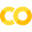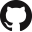# PyTorch Quickstart¶Run in Google ColabView source on GitHub

In this guide we will describe how to scale out PyTorch programs using Orca in 4 simple steps.

## Step 0: Prepare Environment¶

Conda is needed to prepare the Python environment for running this example. Please refer to the install guide for more details.

```conda create -n py37 python=3.7  # "py37" is conda environment name, you can use any name you like.
conda activate py37
pip install bigdl-orca
pip install torch==1.7.1 torchvision==0.8.2
pip install six cloudpickle
pip install jep==3.9.0
```

## Step 1: Init Orca Context¶

```from bigdl.orca import init_orca_context, stop_orca_context

cluster_mode = "local"
if cluster_mode == "local":  # For local machine
init_orca_context(cores=4, memory="10g")
elif cluster_mode == "k8s":  # For K8s cluster
init_orca_context(cluster_mode="k8s", num_nodes=2, cores=2, memory="10g", driver_memory="10g", driver_cores=1)
elif cluster_mode == "yarn":  # For Hadoop/YARN cluster
init_orca_context(
cluster_mode="yarn", cores=2, num_nodes=2, memory="10g",
driver_memory="10g", driver_cores=1,
conf={"spark.rpc.message.maxSize": "1024",
"spark.driver.extraJavaOptions": "-Dbigdl.failure.retryTimes=1"})
```

This is the only place where you need to specify local or distributed mode. View Orca Context for more details.

Note: You should `export HADOOP_CONF_DIR=/path/to/hadoop/conf/dir` when running on Hadoop YARN cluster. View Hadoop User Guide for more details.

## Step 2: Define the Model¶

You may define your model, loss and optimizer in the same way as in any standard (single node) PyTorch program.

```import torch
import torch.nn as nn
import torch.nn.functional as F

class LeNet(nn.Module):
def __init__(self):
super(LeNet, self).__init__()
self.conv1 = nn.Conv2d(1, 20, 5, 1)
self.conv2 = nn.Conv2d(20, 50, 5, 1)
self.fc1 = nn.Linear(4*4*50, 500)
self.fc2 = nn.Linear(500, 10)

def forward(self, x):
x = F.relu(self.conv1(x))
x = F.max_pool2d(x, 2, 2)
x = F.relu(self.conv2(x))
x = F.max_pool2d(x, 2, 2)
x = x.view(-1, 4*4*50)
x = F.relu(self.fc1(x))
x = self.fc2(x)
return F.log_softmax(x, dim=1)

model = LeNet()
model.train()
criterion = nn.NLLLoss()
```

## Step 3: Define Train Dataset¶

You can define the dataset using standard Pytorch DataLoader.

```import torch
from torchvision import datasets, transforms

torch.manual_seed(0)
dir='./'

batch_size=64
test_batch_size=64
transform=transforms.Compose([
transforms.ToTensor(),
transforms.Normalize((0.1307,), (0.3081,))
])),
batch_size=batch_size, shuffle=True)
datasets.MNIST(dir, train=False,
transform=transforms.Compose([
transforms.ToTensor(),
transforms.Normalize((0.1307,), (0.3081,))
])),
batch_size=test_batch_size, shuffle=False)
```

Alternatively, we can also use a Data Creator Function or Orca XShards as the input data, especially when the data size is very large)

## Step 4: Fit with Orca Estimator¶

First, Create an Estimator

```from bigdl.orca.learn.pytorch import Estimator
from bigdl.orca.learn.metrics import Accuracy

est = Estimator.from_torch(model=model, optimizer=adam, loss=criterion, metrics=[Accuracy()])
```

Next, fit and evaluate using the Estimator

```from bigdl.orca.learn.trigger import EveryEpoch

checkpoint_trigger=EveryEpoch())

for r in result:
print(r, ":", result[r])
```

## Step 5: Save and Load the Model¶

Save the Estimator states (including model and optimizer) to the provided model path.

```est.save("mnist_model")
```

Load the Estimator states (model and possibly with optimizer) from the provided model path.

```est.load("mnist_model")
```

Note: You should call `stop_orca_context()` when your application finishes.